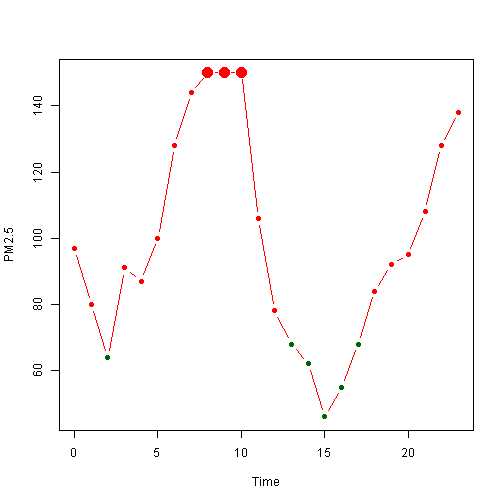``````3 > 2  # 3比2大吗？是的。
``````
``````##  TRUE
``````
``````1 > 2  # 1比2大吗？不是的。
``````
``````##  FALSE
``````
``````!(1 > 2)  # 1不比2大吗？呃......
``````
``````##  TRUE
``````
``````3 > 2 & 1 > 2  # 3比2大，并且1比2大吗？不是的。
``````
``````##  FALSE
``````
``````3 > 2 | 1 > 2  # 3比2大，或者1比2大吗？是的。
``````
``````##  TRUE
``````
``````3 > 2 & !(1 > 2)  # 3比2大，并且1不比2大吗？好像是吧......
``````
``````##  TRUE
``````

``````x <- 1:3
x == 2
``````
``````##  FALSE  TRUE FALSE
``````
``````x == 2 | x == 3
``````
``````##  FALSE  TRUE  TRUE
``````
``````y <- c(4, 1, 2)
x > y
``````
``````##  FALSE  TRUE  TRUE
``````
``````x > y & y > 1
``````
``````##  FALSE FALSE  TRUE
``````
``````练习06.1 设 x <- 6:1，y <- c(3, 5, 7)，判断 x 中的每个元素是否在y中出现。
``````

``````x %in% y
``````
``````##   TRUE  TRUE FALSE
``````

``````x[x %in% y]
``````
``````##  1 2
``````
``````which(x %in% y)
``````
``````##  1 2
``````
``````练习06.2 从 1:100 中挑出既能被 2 整除，又能被 3 整除的数（提示：判断整除的余数是否为零）。
``````

``````pm <- read.csv("c:\\R\\data\\dapengde_DummyR_PM25.csv")
pm\$time[which(pm\$h8 == max(pm\$h8))]
``````
``````##   8  9 10
``````
``````练习06.3 世界卫生组织制订的 PM2.5 指导标准中最宽松的标准是 24 小时均值 75 微克每立方米。请挑出北京一天内超出此标准的时段。
``````

``````x <- 12
if (x < 99) print("x is less than 99")  # 如果()，那么。
``````
``````##  "x is less than 99"
``````
``````
x <- 12
if (x < 99) {
print("x is less than 99")
} else {
print("x is larger than 99")
}  # 如果()，那么{}，否则{}。
``````
``````##  "x is less than 99"
``````
``````
x <- 12
ifelse(x < 99, "small", "large")  # 等同于上一个例子，不同的是这里 x 的向量长度可以大于1，比如试试 x <- 98:100。
``````
``````##  "small"
``````

``````plot(x = pm\$time, y = pm\$h8, xlab = "Time", ylab = "PM2.5", cex = ifelse(pm\$h8 ==
max(pm\$h8), 2, 1), pch = 16, type = "b", col = ifelse(pm\$h8 > 75, "red",
"darkgreen"))
``````## 有用的信息：

( 连载中，待续 )

### 17 Responses to “R 菜鸟入门篇 第06篇 分支”

1. 练习06.1做出的结果是不是有问题啊

• 有什么问题？说来讨论讨论。

• x[x %in% y]的结果应该是5 3吧？

which(x %in% y)的结果应该是2 4吧？

• 很对！

2. R作图很灵活啊，比SAS灵活多了

• 是的，让人爱不释手。

3. 又来打扰了。 最后那幅图，如何改变线的颜色呢？ (比如从红色到蓝色)。 因为符号填充色和线色都是用col 来定义的，有些糊涂了。先谢谢dapeng。

• 可以用一条point()语句添加红色的点，再用另一条point()语句添加蓝色的线。

• 好的，我试试，谢谢啦~

4. 不会用R,但是感觉和PYTHON很像…

Python 做数值计算也不错,而且用途要广泛一些.

• Yihui：R的竞争对手已经不是SAS，而是Python，我对此表示完全同意。如果R核心团队能有更开放的态度，也许还有希望和Python在统计领域一搏，毕竟有强大的先发优势，但如今我们只能面对Python神教的不断奚落去曲线救国，R本身我们动不了，只能从R包入手。

5. **> (6:1)==c(3,5,7)

 FALSE TRUE FALSE TRUE FALSE FALSE**

也可以用==，不需要用%in%?

• 哈哈哈，这个例子太有趣了。试比较 `(1:6) == c(3,5,7)``(1:6) %in% c(3,5,7)`

6. 大鹏，能不能附个练习的答案呢？你有邮箱吗？可以发邮件给你不？

• 答案可以有，但是没必要，而且不重要。可以发邮件给我，dapengde(at)live.com

7. “那三大结构简直就是整个生活的基本结构：日出日落，月圆月缺，年尾年头，这是循环；上学还是就业，单身还是结婚，丁克还是生娃，这是分支；不管是循环还是分支，都嵌入在生老病死的时间轴上，这是顺序。所谓尽人事听天命，想来就是心平气和地接受顺序结构，小心翼翼地制订循环结构，在关键时刻控制好分支结构，就这样度过一生罢”

写的真赞啊。。。把编程上升到了哲学高度了。。。

（14年与先生在德国和奥地利自驾，顺便探访住在萨尔斯堡附近小镇的好朋友，本来是一个R菜鸟在四处搜实用帖，结果被您的生活贴吸引了。非常欣赏您的生活哲学。佩服！）

8. x <- 6:1
y <- c(3,5,7)
x==y

 FALSE TRUE FALSE TRUE FALSE FALSE